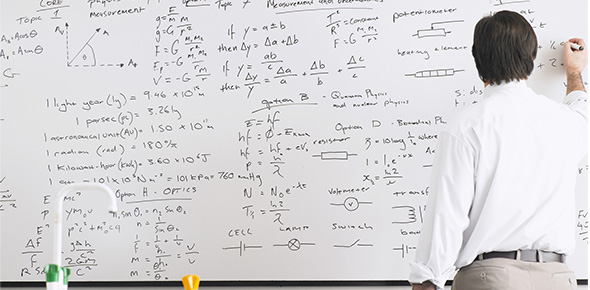# Compounds And Reactions

24 QuestionsSettings.

Related Topics
• 1.
Look at the following chemical equation:2Fe2O3  +  3C  ----->    4Fe  +  3CO2What are the products?
• 2.
Look at the following chemical equation:2Fe2O3  +  3C  ----->    4Fe  +  3CO2What are the reactants?
• 3.
Look at the following chemical equation:2Fe2O3  +  3C  ----->    4Fe  +  3CO2How many Oxygen molecules go into the reaction?
• 4.
Look at the following chemical equation:2Fe2O3  +  3C  ----->    4Fe  +  3CO2How many oxygen molecule are produced in the reaction?
• 5.
Look at the following chemical equation:2Fe2O3  +  3C  ----->    4Fe  +  3CO2What the "+" sign mean between 2Fe2O3  +  3C?
• 6.
Look at the following chemical equation:2Fe2O3  +  3C  ----->    4Fe  +  3CO2What the "+" sign mean between 4Fe  +  3CO2?
• 7.
Look at the following chemical equation:2Fe2O3  +  3C  ----->    4Fe  +  3CO2In the chemical formula, 2Fe2O3 , how many Iron (Fe) atoms are there?
• 8.
Look at the following chemical equation:2NaOH  +  H2SO4  ----->    Na2SO4 +  2H2OWhat are the products?
• 9.
Look at the following chemical equation:2NaOH  +  H2SO4  ----->    Na2SO4 +  2H2OWhat are the reactants?
• 10.
Look at the following chemical equation:2NaOH  +  H2SO4  ----->    Na2SO4 +  2H2OIn the chemical formula 2NaOH, what is the coefficient number?
• 11.
Look at the following chemical equation:2NaOH  +  H2SO4  ----->    Na2SO4 +  2H2OIn the chemical formula Na2SO4, what is the coefficient number?
• 12.
Look at the following chemical equation:2NaOH  +  H2SO4  ----->    Na2SO4 +  2H2OWhat does the arrow mean?
• 13.
Look at the following chemical equation:2NaOH  +  H2SO4  ----->    Na2SO4 +  2H2OIn the chemical formula H2SO4 , How many Sulfur (S) are there?
• 14.
Look at the following chemical equation:2NaOH  +  H2SO4  ----->    Na2SO4 +  2H2OIn the chemical formula H2SO4 , How many Oxygen (O) are there?
• 15.
Look at the following chemical equation:2NaOH  +  H2SO4  ----->    Na2SO4 +  2H2OIn the chemical formula 2H2O , How many Hydrogen (H) are there?
• 16.
What forms when two or more substances combine chemically?
• 17.
What is the shorthand way of writing a compound called?
• 18.
What is the shorthand way of writing a chemical reaction called?
• 19.
What is the name of the Law that states matter can not be created nor destroyed?
• 20.
What is the name given to the electrons that do the bonding or reacting in an atom?
• 21.
Where are valence electrons located?
• 22.
Where are the protons and neutrons located in an atom?
• 23.
How do you solve for neutrons?
• 24.
How do you find the number of electrons and protons in an atom?